# Class Circle

Calculation of the properties of circles

## Description

The class Circle calculates properties of a circle.

The default property is the radius. This means that the radius $$r$$ can be passed as an argument without a command string (see example below).

## Properties

The following properties can be queried as a result or passed as an argument.

#### Description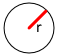r Radius (default)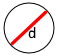d Diameter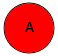A Area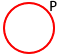P Perimeter

## Syntax

Object = Circle (radius)

Object = Circle ("args names", arguments)

Value = Circle ("get –> args names", arguments)

## Examples

The following example specifies a radius of 5.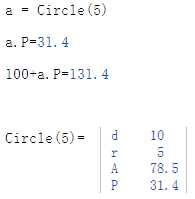In the following example, area $$A$$ is calculated from a radius of 5.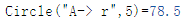The following example calculates the properties from a diameter of 10.